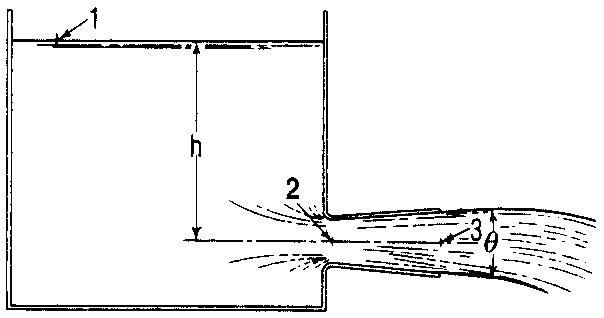Search

# Orifice Discharge Into Diverging Conical Tubes

Posted in Hydraulics |Email This Post |

This type of tube can greatly increase the flow through an orifice by reducing the pressure at the orifice below atmospheric. The formula that follows for the pressure at the entrance to the tube is obtained by writing the Bernoulli equation for points 1 and 3 and points 1 and 2P2=wh[1-( a3/ a2 )*( a3/ a2 ) ]

where

p2= gage pressure at tube entrance, lb/ft2 (Pa)

w =unit weight of water, lb/ft3 (kg/m3)

h =head on centerline of orifice, ft (m)

a2 =area of smallest part of jet (vena contracta, if one exists), ft2 (m)
a3 =area of discharge end of tube, ft2 (m2)

Discharge is also calculated by writing the Bernoulli equation for points 1 and 3.
For this analysis to be valid, the tube must flow full, and the pressure in the throat of the tube must not fall to the vapour pressure of water. Experiments by Venturi show the most efficient angle to be around 5°.

More Entries :